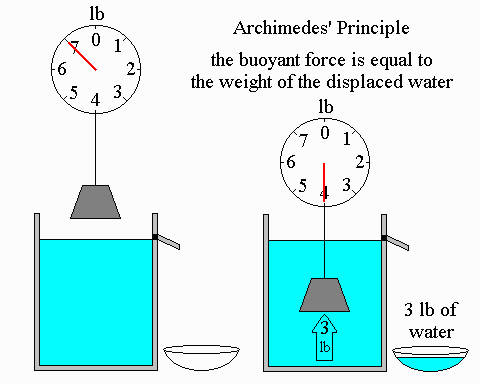# CBSE Class 9 Science Chapter 10 Revision Notes

Chapter 10: Gravitation Revision Notes

• Gravity is one of the universe’s most fundamental forces. It is crucial not just to the structure of our solar system, but also to the behavior of things on Earth.

Gravitation

• The attraction between two things in the universe is known as gravity. The attraction of items by the earth is known as gravitation.
• This force is proportional to the product of the objects’ masses and inversely proportional to the square of their separation. It is independent of medium.

The force of gravity

• For example, if a body is thrown from a specific height, gravity causes it to fall downhill. When a body is flung aloft, it reaches a specific height before falling back down owing to gravity.
• Gravitation might be the attraction that exists between things in space.
• For example, the earth and the moon are attracted to each other.
• The sun and the planets are attracted to each other.
• The moon is kept in orbit by a natural force that pushes all things toward the earth’s centre.
• It binds the stars together…
• And it connects galaxies for billions of years….
• Keeps planets’ atmospheres from evaporating.

The Universal Law of Gravitation

• According to Newton’s Law of Gravitation, every item in the universe attracts every other object with a force that is directly proportional to the product of their masses and inversely proportional to the square of their separation.

The Moon’s Falling – Moon’s revolution around Earth

• The centripetal force, which is the Earth’s gravitational pull, causes the moon to rotate around it.
• The moon will continue to move on a straight-line path tangential to its orbit around the Earth if the force of attraction between the Earth and the moon ends.

Centripetal force

• When a body moves in a circular motion, it encounters a force that acts towards the circle’s centre.
• A centripetal force is a force that seeks the centre.

Motion and Free Fall

• The acceleration due to gravity remains constant at g =9.8 ms (raise to -2) when an item is in free fall
• The inertia of an item is measured by its mass, which is constant throughout the universe. As the value of g changes, so does the weight of an item. Weight is nothing more than the Earth’s gravitational pull on an item = mg.
• An item’s weight on the Moon is 1/6 that of an object on Earth.

Pressure & Thrust

• Thrust is the force acting perpendicular to the surface on an object.
• The force acting on a unit area of a surface is known as pressure.
• The SI unit is pascal.
• When a force is applied to a smaller area, it exerts more pressure than when the same force is applied to a wider area.

Pressure In Fluids

• Pressure is exerted by fluids in all directions.
• The pressure applied to fluids is distributed evenly in all directions.

Buoyancy

• When an item is submerged in a fluid, it experiences buoyant force, which causes it to rise.
• This is referred to as buoyancy or upthrust.

Why do objects float or sink when placed on the surface of water?

• Fill a beaker halfway with water. Take a piece of cork and a similar-mass iron nail. Toss them into the sea. The nail sinks and the cork floats.
• If the density of an object is less than that of a liquid, it will float in the liquid, but if the density of an object is more than that of a liquid, it will sink.

Archimedes PrincipleSource:

• When a body is partially or entirely immersed in a fluid, it experiences an upward force proportional to the weight of the fluid displaced by it, according to Archimedes’ principle.

Relative density

• The ratio of a substance’s density to the density of water is known as relative density. It’s a proportion of similar amounts that doesn’t have a unit.
• The value of g is independent of mass, meaning that in free fall, any object, large or tiny, feels the same acceleration due to gravity. For freely falling objects, all three equations of motion are true, just as they are for uniform motion.
• The convention sign is +ve while facing earth g and -ve when facing away from earth g.
]]>